# Use KVL to determine values of branch currents

• DevonZA
Fortunately, you've already calculated those. You should be able to take it from there.I1=I2+I3=0.015+0.013=0.028AWhich is the same as the answer in my study guide. So I5 is not needed to be calculated?In summary, the conversation discusses solving a circuit problem using Kirchhoff's Voltage Law (KVL). The first attempt at a solution is incorrect as the voltage across a 12 ohm resistor is assumed to be 9 volts, which is not the case. The correct approach involves assigning currents to all branches and using KVL to solve for the unknown

## Homework Statement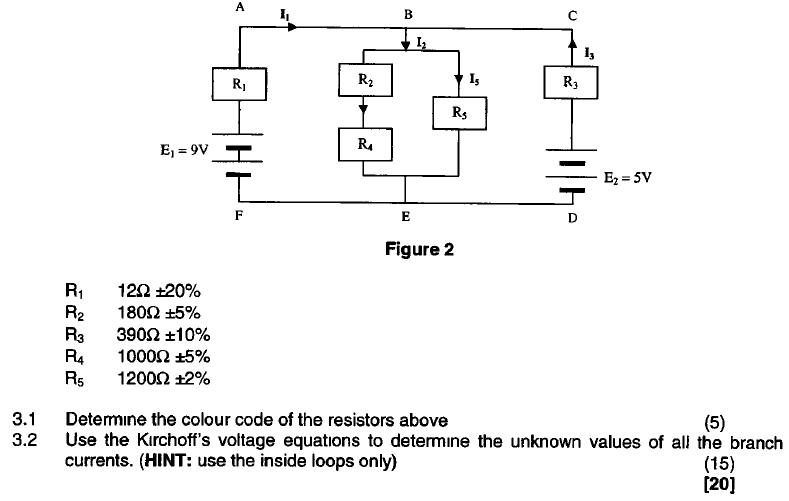## Homework Equations

KVL: At any instant in a closed loop the algebraic sum of the e.m.f's acting round the loop is equal to the algebraic sum of the p.d's round the loop.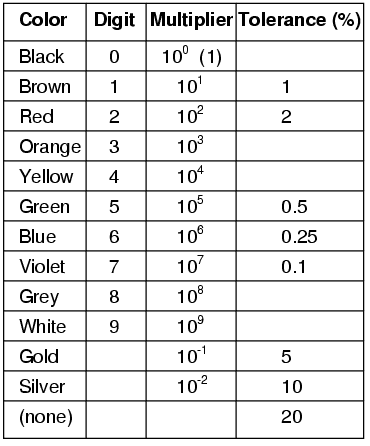## The Attempt at a Solution

3.1
R1 = BROWN, RED, BLACK, NONE
R2 = BROWN, GREY, BROWN, SILVER
R3 = ORANGE, WHITE, BROWN, GOLD
R4 = BROWN, BLACK, RED, SILVER
R5 = BROWN. RED, RED, RED

3.2
I want to add R2 and R4 because they are in series. 180ohms + 1000ohms = 1180ohms
The R2+R4 are in parallel with R5. (1/1180+1/1200)^-1=594.96ohms

Using KVL:
V=IR
9=I1x12
I1=9/12=0.75A

I2=9/594.96=0.015A

I3=5/390=0.013A

Okay so I'm pretty sure this is not correct but the middle area with R2, R4 and R5 is really confusing me.
Why is there I5 when we already have current I2?
Also I assume I4 is the little arrow indicated above R4 but by that reasoning then there should be a current above R2 as well?

DevonZA said:

## Homework Statement

View attachment 100104

## Homework Equations

KVL: At any instant in a closed loop the algebraic sum of the e.m.f's acting round the loop is equal to the algebraic sum of the p.d's round the loop.

View attachment 100105

## The Attempt at a Solution

3.1
R1 = BROWN, RED, BLACK, NONE
R2 = BROWN, GREY, BROWN, SILVER
R3 = ORANGE, WHITE, BROWN, GOLD
R4 = BROWN, BLACK, RED, SILVER
R5 = BROWN. RED, RED, RED

3.2
I want to add R2 and R4 because they are in series. 180ohms + 1000ohms = 1180ohms
The R2+R4 are in parallel with R5. (1/1180+1/1200)^-1=594.96ohms

Using KVL:
V=IR
9=I1x12
I1=9/12=0.75A

This is incorrect. The voltage across the 12 ohms is not 9 volts. You are not using kvl here. You must apply lvl: assign currents to all the branches, pick a loop and go around it, adding all the voltages.

I1-I2+I3=0
I3=I2-I1
Loop ABEFA:
+9+12I1+594.96I2=0

Loop BCDEB:
-5v-594.96I5-390I3=0
-5-594.96I5-390(I2-I1)=0
-5-594.96I5-390I2+390I1

Loop ABEFA x32.5
292.5+390I1+19336.20I2=0
Loop BCDEB
-5-594.96I5-390I2+390I1

Subtract Loop BCDEB from loop ABEFA
297.5-594.96I5+19726.2I2=0

This I5 current is killing me

DevonZA said:
I1-I2+I3=0
I3=I2-I1
Loop ABEFA:
+9+12I1+594.96I2=0

Loop BCDEB:
-5v-594.96I5-390I3=0
-5-594.96I5-390(I2-I1)=0
-5-594.96I5-390I2+390I1

Loop ABEFA x32.5
292.5+390I1+19336.20I2=0
Loop BCDEB
-5-594.96I5-390I2+390I1

Subtract Loop BCDEB from loop ABEFA
297.5-594.96I5+19726.2I2=0

This I5 current is killing me
Watch your signs. You need to decide if you're summing potential rises or potential drops as positive values.

When you "walk" the first loop clockwise, the 9 V source is definitely a potential rise but walking through R1 you are traveling in the same direction as its current I1, so there must be a potential drop across that resistor. So both terms cannot have the same sign in your loop equation: one is a rise and the other a drop.

You have combined R2, R4, and R5 into a single resistance value (594.96 Ω) and yet you're writing loop equations with the individual resistors. That's why I5 is showing up to bother you. Only I2 flows through the equivalent resistance.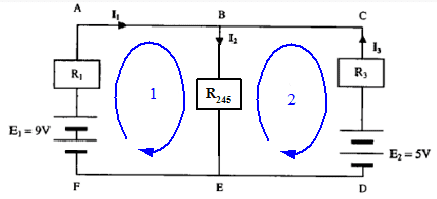You can go back and solve for I5 after you have a value for I2.

Thank you gneill.
Just to clarify:
The polarity landed on when traveling through a battery is the sign that must be used. So I'm this case we are traveling clockwise through E1 thus landing on the positive terminal =+9v.

When traveling through a resistor in the same direction as current flow then the sign is positive. So in this case when traveling through R1 the sign is positive?

I will attempt the problem again using what you've advised and I will revert back.

Thanks again.

Or am I confused?

DevonZA said:
Just to clarify:
The polarity landed on when traveling through a battery is the sign that must be used. So I'm this case we are traveling clockwise through E1 thus landing on the positive terminal =+9v.

The battery symbol has the implied polarity: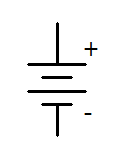Loop ABEFA
+9v+12I1+594.96I2=0

Loop BCDEB
-5-594.96I2-390I3=0
-5-594.96I2-390(I2-I1)=0
-5+390I1-984.96I2=0

Loop ABEFA x 32.5
292.5+390I1+19336.2I2=0
Subtract Loop BCDEB
-5+390I1-984.96I2=0
Gives:
297.5+20321.16I2=0
I2=-0.015A (opposite direction)

Substitute I2 into loop ABEFA
9+12I1+594.96(0.015)=0
I1=-1.49A (opposite direction)

I believe my signs are correct according to my study guide?
If so how do I find I5?
It looks to me as though the tip of the arrow of I2 is a junction but then I would need a third current?

DevonZA said:
I believe my signs are correct according to my study guide?
No, the signs of your voltage source terms are not correct. You are apparently summing potential drops (judging by the signs of your resistor&current terms), so "walking" through the 9 V battery in a clockwise fashion is a potential rise of 9 V, which means it is a "drop" of -9 V.
DevonZA said:
If so how do I find I5?
It looks to me as though the tip of the arrow of I2 is a junction but then I would need a third current?
Once you have I2 you can isolate the R2-R4-R5 subcircuit and determine the currents in its two paths. There are a few choices of method to do so including using the current division rule or the common potential difference that is across the two.

I was doing emf and volt drops equal to zero. Okay let me try again.

I get I2=0.015A
I1=0.0063A

Using current divider rule:
I5=(R2/R2+R5)xI2
=0.00196A

I4=(R2/R2+R4)xI2
=0.00229A

DevonZA said:
I get I2=0.015A
I1=0.0063A
I2 is okay assuming it's rounded to two sig figs. I1 does not look right.

DevonZA said:
Using current divider rule:
I5=(R2/R2+R5)xI2
=0.00196A
That's not the correct way to apply the current divider rule. One path has R5 in it but the other has both R2 and R4 in series. You need to use the total resistances of the paths.

What are your concerns for I1?

I5=(180/1180+1200)x0.015
=0.0013A

I assume you are happy with the way I calculated I4? Or at least where I think I4 is

DevonZA said:
What are your concerns for I1?
Your value of 6.3 mA is not correct. I suspect that you're still using incorrect signs for the voltage sources in your KVL equations.
I5=(180/1180+1200)x0.015
=0.0013A
One branch has a total of 1180 Ω, the other 1200 Ω. The current division rule uses the total resistances in its equation.
Note that you should use parentheses to make the order of operations unambiguous:

I5 = (1180/(1180 + 1200) )xI2
I assume you are happy with the way I calculated I4? Or at least where I think I4 is
Nope. You've not applied current division correctly for the two paths that I2 splits into.

Edit: Here's a diagram that portrays the situation with its equivalent simplified form on the right: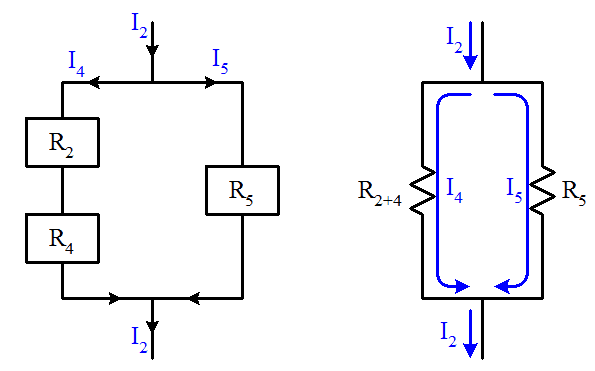Last edited:
•cnh1995
Thanks again for your assistance gneill.

Substitute I2 into equation 1:
12I1+594.96(0.015)=-9
12I1=-17.9244
I1=-1.4937A

nope that can't be right.

I5 = (1180/(1180 + 1200) )xI2
= 0.0074A

I4=R5/R2+R4 +R5 x I2
=(1200)/(1180+1200) x 0.015
= 0.0076A

Let's step back and take a fresh run at the problem. I think you're relying on equations that haven't been vetted.

Taking the version of the circuit (from post #4) where the central resistors have been reduced to a single value (594.958 Ω):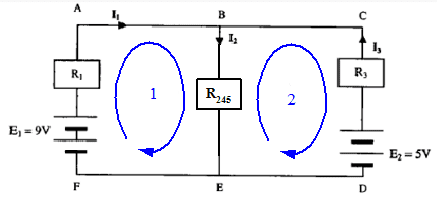Write the two KVL loop equations and the KCL equation for the junction at point B. You can write them symbolically for now; forget plugging in numbers for now.

gneill said:
Let's step back and take a fresh run at the problem. I think you're relying on equations that haven't been vetted.

Taking the version of the circuit (from post #4) where the central resistors have been reduced to a single value (594.958 Ω):Write the two KVL loop equations and the KCL equation for the junction at point B. You can write them symbolically for now; forget plugging in numbers for now.

Loop 1 analyzing in a clockwise direction:
emf's = +9V
voltdrops = +I1R1+I2R245
equation: 9=I1R1+I2R245

Loop 2 analyzing in a clockwise direction:
emf's = -5V
voltdrops = -I2R245-I3R3
equation: -5=-I2R245-I3R3

I am using the exact same method as found in my study guide

DevonZA said:

Loop 1 analyzing in a clockwise direction:
emf's = +9V
voltdrops = +I1R1+I2R245
equation: 9=I1R1+I2R245
Good for loop 1.
Loop 2 analyzing in a clockwise direction:
emf's = -5V
voltdrops = -I2R245-I3R3
equation: -5=-I2R245-I3R3
Not so good for loop 2. Note the directions that I2 and I3 are defined to flow through the resistors. You are KVL-walking against those flows as you go around the loop clockwise. So rather than encountering potential drops, you're passing through potential rises there.

Rewrite the equation for loop two taking this into account, and then write the KCL equation for junction B.

Edit: Hold on, I just realized that I made an error in assessing your work!I wrote the equations summing rises rather than drops and failed to "translate" the signs properly to compare with yours.

Your two equations are both fine. Can you write the KCL equation to give you the required third equation?

Analyzing clockwise:
We are traveling through R245 against the current I2 which according to my study guide means that we get -I2R245
We are traveling through R3 against the current I3 which according to my study guide means that we get -I3R3

I'm sorry I really don't understand, I am not trying to be funny.

At Junction B according to KCL: I1-I2+I3=0 or I3=I2-I1

DevonZA said:
Analyzing clockwise:
We are traveling through R245 against the current I2 which according to my study guide means that we get -I2R245
We are traveling through R3 against the current I3 which according to my study guide means that we get -I3R3

I'm sorry I really don't understand, I am not trying to be funny.

At Junction B according to KCL: I1-I2+I3=0 or I3=I2-I1
You're right and I apologize. It was my error. I updated my post but you clearly got to it before I'd finished the update.

You've got three correct equations with which you can solve for the three currents. Can you show that?

gneill said:
You're right and I apologize. It was my error. I updated my post but you clearly got to it before I'd finished the update.

You've got three correct equations with which you can solve for the three currents. Can you show that?

No need to apologize, I really appreciate your help.
First time I've been right though :)

9=I1R1+I2R245
9=I1(12)+I2(594.96) ...1

-5=-I2R245-I3R3
-5=-I2(594.96)-I3(390) but I3=I2-I1
-5=-I2(594.96)-(390)(I2-I1)
-5=+I1(390)-I2(984.96) ...2

9=I1(12)+I2(594.96) x 32.5
= 292.5=I1(390)+I2(19336.2)
-5=+I1(390)-I2(984.96) -

= 297.5=I2(20231.16)
I2=0.015A

Sub into 1:
9=I1(12)+(0.015)(594.96)
I1(12)=0.0756
I1=0.0063A

I3=I2-I1
I3=0.015-0.0063
I3=0.0087A

DevonZA said:
No need to apologize, I really appreciate your help.
First time I've been right though :)

9=I1R1+I2R245
9=I1(12)+I2(594.96) ...1

-5=-I2R245-I3R3
-5=-I2(594.96)-I3(390) but I3=I2-I1
-5=-I2(594.96)-(390)(I2-I1)
-5=+I1(390)-I2(984.96) ...2

9=I1(12)+I2(594.96) x 32.5
= 292.5=I1(390)+I2(19336.2)
-5=+I1(390)-I2(984.96) -
= 297.5=I2(20231.16)
I2=0.015A
You should keep a few more digits in your intermediate value for I2 since it is going to be used for further calculations. Here it's rounded to two significant figures, when a better value for keep at least two more digits: 0.01464. That's 14.64 mA. The next bit where you are substituting for I2 results in a difference between similarly sized values, and those significant figures will be, well significant!
Sub into 1:
9=I1(12)+(0.015)(594.96)
I1(12)=0.0756
I1=0.0063A
This value of I1 is off by quite a bit due to loss of accuracy (early rounding of intermediate values). Try again keeping more digits in the value of I2.

Sub into 1:
9=I1(12)+(0.01464)(594.96)
I1(12)=0.2898
I1=0.02415A

Wow that is significant!

DevonZA said:
Sub into 1:
9=I1(12)+(0.01464)(594.96)
I1(12)=0.2898
I1=0.02415A

Wow that is significant!
Yup. Make a habit of not rounding anything (except for presentation) until the very end.

Once you've squared away accurate values for I1 and I3, and using your new improved accuracy I2, tackle the current division in the central network:gneill said:
Yup. Make a habit of not rounding anything (except for presentation) until the very end.

Will do, thanks again for your help.
Now to find I4 and I5 :

Is this correct
I5 = (1180/(1180 + 1200) )xI2
= 0.0074A

I4=R5/R2+R4 +R5 x I2
=(1200)/(1180+1200) x 0.015
= 0.0076A

EDIT - oops I was too quick to ask another question.

gneill said:
Once you've squared away accurate values for I1 and I3, and using your new improved accuracy I2, tackle the current division in the central network: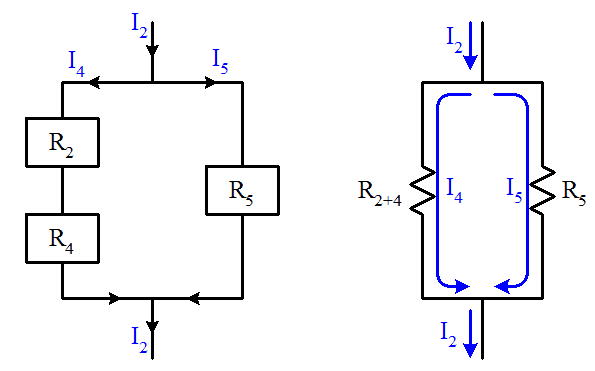Right so I3=I2-I1
I3=0.015-0.02415
I3=-0.00915A (negative value indicates current flows in opposite direction?)

Before I continue, I need a voltage value right? Would that be 12V?

Sorry gneill I am asking silly questions.
I missed your point about using current division, apologies it is getting late here.

I4=((R5)/(R2+R4))xI2
= (1200/1180)x0.015
= 0.01525A

I5=((R2+R4)/(R5)xI2
= (1180/1200)x0.015
= 0.01475A

Okay I need to sleep, will check back in the morning.
Thanks again gneill really appreciate your time and effort in assisting me.

DevonZA said:
Sorry gneill I am asking silly questions.
I missed your point about using current division, apologies it is getting late here.
No, it's a valid approach to first find the potential across the middle subcircuit and then find the currents through its parallel branches using Ohm's law. In fact I'd recommend doing so for a check on the current division results.

To find the potential difference across the middle subcircuit, that is the potential between points B and E, do a KVL walk from E to B either through the E1 path or the E2 path. You know the currents I1 and I3 so you can apply Ohm's law to find the drops across resistors R1 or R3. Beware of actual current directions.

A note on current division:

When you have two parallel paths with resistances ##R_a## and ##R_b## and an entering current ##I##, the individual path currents are given by ##I## multiplied by resistance of the "other" path divided by the sum of the two path resistances. So for example:

##I_a = I \frac{R_b}{R_a + R_b}##

You should prove this property to your satisfaction so that it becomes second nature to apply it, and so you can quickly re-derive it should you forget